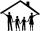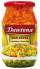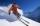# Pytagoriade

Two fifth-graders teams competing in math competitions - in Mathematical Olympiad and Pytagoriade. Of the 33 students competed in at least one of the contest 22 students. Students who competed only in Pytagoriade was twice more than those who just competed in the Mathematical Olympiad. Students who competed in both competitions was 4 times more than those who only competed in Pytagoriade.

a) How many students competed in both competitions?
b) How many students competed in just one competition?
c) How many students competed in no more than one competition?

Result

a =  16
b =  6
c =  17

#### Solution:

p+a+o = 22
p = 2 o
a = 4p
b = p+o
c = 33-a

a+o+p = 22
2o-p = 0
a-4p = 0
b-o-p = 0
a+c = 33

a = 16
b = 6
c = 17
o = 2
p = 4

Calculated by our linear equations calculator.

Leave us a comment of example and its solution (i.e. if it is still somewhat unclear...):

Showing 0 comments:Be the first to comment!#### To solve this verbal math problem are needed these knowledge from mathematics:

Do you have a linear equation or system of equations and looking for its solution? Or do you have quadratic equation?

## Next similar examples:

1. Brothers and sistersLenka has as many brothers as sisters. Her brothers have twice as many sisters as brothers. How many brothers and sisters are in the family?
2. Stamps and albumsMichail has in three albums stored 170 stamps. In the first album, there are 14 more than in the second and in the second is by 1/5 less than in the third. How many stamps are in the first album?
3. Belgium vs ItalyBelgium played a match with Italy and Belgium win by 2 goals. The match fell a total 6 goals. Determine the number of goals scored by Belgium and by Italy.
4. Box of chocolatesIn a box of chocolates was 16 candies. Christopher and Luke they were distributed: a) Christopher had about 4 candies over Luke, b) Christopher had about 6 candies less than Luke, c) Christopher had 3 times more sweet than Luke. How many had each boy can
5. AddendsNumber 118 divide into two addends, so first addend is 69 greater than 75% of the second addend.
6. FamiliesIn class we investigated how many children have family in which its live. How many% of pupils live in families with more than two children? If five families each have one child in 9 families have 2 children, in 5 families have 3 children, in 2 families ha
7. Vijuviju has 40 chickens and rabbits. If in all there are 90 legs. How many rabbits are there with viju??
8. The storeThe store received the same number of cans of peas and corn. The first day sold 10 cans of peas and 166 cans ofcorn so that left 5 times more peas than corn cans. How many cans of each kind were in the store?
9. Three brothersThe three brothers have a total of 42 years. Jan is five years younger than Peter and Peter is 2 years younger than Michael. How many years has each of them?
10. Ski lessonsOn the ski course leaves 80 pupils. They live in triple and quadruple rooms. Count if rooms is the 23. How many are triple and quadruple rooms?
11. One-thirdA one-third of unknown number is equal to five times as great as the difference of the same unknown number and number 28. Determine the unknown number.
12. FactoryIn the factory workers work in three shifts. In the first inning operates half of all employees in the second inning and a third in the third inning 200 employees. How many employees work at the factory?
13. Unknown number 11That number increased by three equals three times itself?
14. CandyPeter had a sachet of candy. He wanted to share with his friends. If he gave them 30 candies, he would have 62 candies. If he gave them 40 candies, he would miss 8 candies. How many friends did Peter have?
15. Magic numberThe number 135 split to two addends so that one addend was 30 greater than 2/5 the addend.
16. Three workersThree workers were rewarded CZK 9200 and the money divided by the work they have done. First worker to get twice than the second, the second three times more than the third. How much money each worker received?
17. Substitutionsolve equations by substitution: x+y= 11 y=5x-25